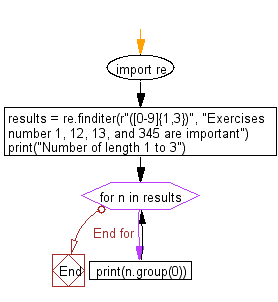﻿ Python Exercises: Search the numbers of length between 1 to 3 in a given string - w3resource# Python: Search the numbers (0-9) of length between 1 to 3 in a given string

## Python Regular Expression: Exercise-18 with Solution

Write Python program to search the numbers (0-9) of length between 1 to 3 in a given string.

Sample Solution:-

Python Code:

``````import re
results = re.finditer(r"([0-9]{1,3})", "Exercises number 1, 12, 13, and 345 are important")
print("Number of length 1 to 3")
for n in results:
print(n.group(0))
```
```

Sample Output:

```Number of length 1 to 3
1
12
13
345
```

Flowchart:## Visualize Python code execution:

The following tool visualize what the computer is doing step-by-step as it executes the said program:

Python Code Editor:

Have another way to solve this solution? Contribute your code (and comments) through Disqus.

What is the difficulty level of this exercise?

﻿

New Content: Composer: Dependency manager for PHP, R Programming